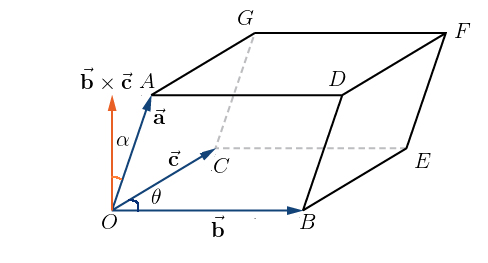Filter By

## All Questions

#### Let the volume of a parallelopiped whose coterminous edges are given byandbe 1 cu. unit. Ifbe the angle between the edgesandthencan be : Option: 1Option: 2Option: 3Option: 4Dot (Scalar) Product in Terms of Components -

Angle between two vectors-

Geometrical Interpretation of Scalar Triple Product -

Let vectorsandrepresented the sides of a parallelepiped OA, OB and OC respectively. Then,is a vector perpendicular to the plane ofand. Let ? be the angle between vectorsandand α be the angle betweenand.

Ifis a unit vector along, then α is the angle betweenand.-Correct Option (3)

#### Letandbe two vectors. Ifis a vector such thatandthenis equal to : Option: 1Option: 2Option: 3Option: 4Vector Triple Product -

For three  vectorsandvector triple product is defined as.-Correct Option (3)

## Crack CUET with india's "Best Teachers"

• HD Video Lectures
• Unlimited Mock Tests
• Faculty Support#### Letandbe three unit vectors such that.Ifandthen the ordered pair,is equal to : Option: 1Option:2Option: 3Option: 4Addition and subtraction of Vectors -

The sum of two vectors is always a vector.Properties of vector Subtraction-Correct Option (3)

#### Letbe three mutually perpendicular vectors of the same magnitude and equally inclined at an angle, with the vector. Thenis equal to ________.

Given,

and these are mutually perpendicular,

So,

Angle of with

Now,

Using this in (i)

Hence, the correct answer is 4

## Crack NEET with "AI Coach"

• HD Video Lectures
• Unlimited Mock Tests
• Faculty Support#### Let. Let a vectorbe in the plane containing. Ifis perpendicular to the vectorand its projection onis 19 units, thenis equal to___________

(a vector in the plane of & and perpendicular  to )

#### Letbe three vectors mutually perpendicular to each other and have same magnitude. If a vectorsatisfiesthenis equal to :Option: 1Option: 2Option: 3Option: 4Give expression is

## Crack JEE Main with "AI Coach"

• HD Video Lectures
• Unlimited Mock Tests
• Faculty SupportOR
option (4)

#### The angle between the straight lines, whose direction cosines are given by the equations, is :Option: 1Option: 2Option: 3Option: 4...............(1)

..........(2)

From (1) and (2)

So

Also

So

Angle

## Crack CUET with india's "Best Teachers"

• HD Video Lectures
• Unlimited Mock Tests
• Faculty Support#### If the projection of the vectoron the sum of the two vectorsandis 1, thenis equal to ________.

Sum of vectors (  ) is

Projection of on is